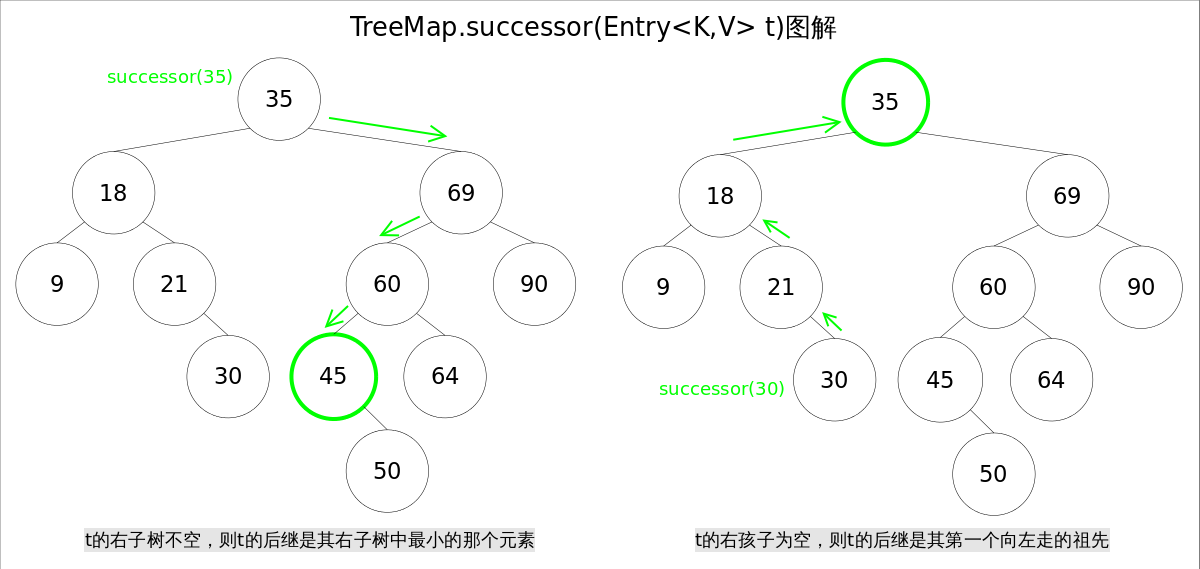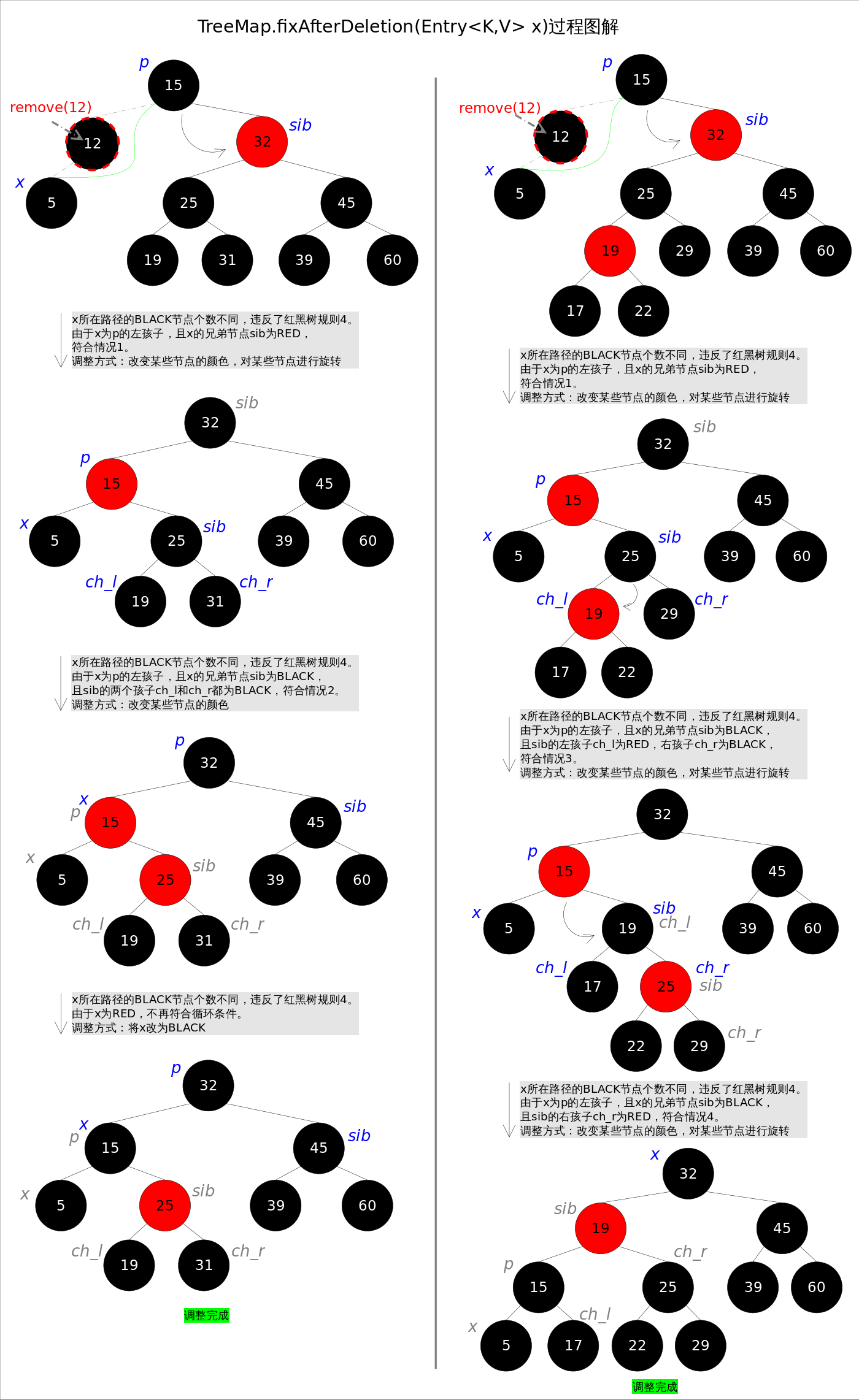# 寻找节点后继

1. t 的右子树不空，则 t 的后继是其右子树中最小的那个元素。
2. t 的右孩子为空，则 t 的后继是其第一个向左走的祖先。TreeMap中寻找节点后继的代码如下：

// 寻找节点后继函数successor()
static <K,V> TreeMap.Entry<K,V> successor(Entry<K,V> t) {
if (t == null)
return null;
else if (t.right != null) {// 1. t的右子树不空，则t的后继是其右子树中最小的那个元素
Entry<K,V> p = t.right;
while (p.left != null)
p = p.left;
return p;
} else {// 2. t的右孩子为空，则t的后继是其第一个向左走的祖先
Entry<K,V> p = t.parent;
Entry<K,V> ch = t;
while (p != null && ch == p.right) {
ch = p;
p = p.parent;
}
return p;
}
}


## remove()

remove(Object key)的作用是删除key值对应的entry，该方法首先通过上文中提到的getEntry(Object key)方法找到key值对应的entry，然后调用deleteEntry(Entry<K,V> entry)删除对应的entry。由于删除操作会改变红黑树的结构，有可能破坏红黑树的约束条件，因此有可能要进行调整。

getEntry()函数前面已经讲解过，这里重点放deleteEntry()上，该函数删除指定的entry并在红黑树的约束被破坏时进行调用fixAfterDeletion(Entry<K,V> x)进行调整。

1. 删除点p的左右子树都为空，或者只有一棵子树非空。
2. 删除点p的左右子树都非空。

// 红黑树entry删除函数deleteEntry()
private void deleteEntry(Entry<K,V> p) {
modCount++;
size--;
if (p.left != null && p.right != null) {// 2. 删除点p的左右子树都非空。
Entry<K,V> s = successor(p);// 后继
p.key = s.key;
p.value = s.value;
p = s;
}
Entry<K,V> replacement = (p.left != null ? p.left : p.right);
if (replacement != null) {// 1. 删除点p只有一棵子树非空。
replacement.parent = p.parent;
if (p.parent == null)
root = replacement;
else if (p == p.parent.left)
p.parent.left  = replacement;
else
p.parent.right = replacement;
p.left = p.right = p.parent = null;
if (p.color == BLACK)
fixAfterDeletion(replacement);// 调整
} else if (p.parent == null) {
root = null;
} else { // 1. 删除点p的左右子树都为空
if (p.color == BLACK)
fixAfterDeletion(p);// 调整
if (p.parent != null) {
if (p == p.parent.left)
p.parent.left = null;
else if (p == p.parent.right)
p.parent.right = null;
p.parent = null;
}
}
}


1.改变某些节点的颜色

2.对某些节点进行旋转。a) 如果是由情况1之后紧接着进入的情况2，那么情况2之后一定会退出循环（因为x为红色）；b) 一旦进入情况3和情况4，一定会退出循环（因为x为root）。

private void fixAfterDeletion(Entry<K,V> x) {
while (x != root && colorOf(x) == BLACK) {
if (x == leftOf(parentOf(x))) {
Entry<K,V> sib = rightOf(parentOf(x));
if (colorOf(sib) == RED) {
setColor(sib, BLACK);                   // 情况1
setColor(parentOf(x), RED);             // 情况1
rotateLeft(parentOf(x));                // 情况1
sib = rightOf(parentOf(x));             // 情况1
}
if (colorOf(leftOf(sib))  == BLACK &&
colorOf(rightOf(sib)) == BLACK) {
setColor(sib, RED);                     // 情况2
x = parentOf(x);                        // 情况2
} else {
if (colorOf(rightOf(sib)) == BLACK) {
setColor(leftOf(sib), BLACK);       // 情况3
setColor(sib, RED);                 // 情况3
rotateRight(sib);                   // 情况3
sib = rightOf(parentOf(x));         // 情况3
}
setColor(sib, colorOf(parentOf(x)));    // 情况4
setColor(parentOf(x), BLACK);           // 情况4
setColor(rightOf(sib), BLACK);          // 情况4
rotateLeft(parentOf(x));                // 情况4
x = root;                               // 情况4
}
} else { // 跟前四种情况对称
Entry<K,V> sib = leftOf(parentOf(x));
if (colorOf(sib) == RED) {
setColor(sib, BLACK);                   // 情况5
setColor(parentOf(x), RED);             // 情况5
rotateRight(parentOf(x));               // 情况5
sib = leftOf(parentOf(x));              // 情况5
}
if (colorOf(rightOf(sib)) == BLACK &&
colorOf(leftOf(sib)) == BLACK) {
setColor(sib, RED);                     // 情况6
x = parentOf(x);                        // 情况6
} else {
if (colorOf(leftOf(sib)) == BLACK) {
setColor(rightOf(sib), BLACK);      // 情况7
setColor(sib, RED);                 // 情况7
rotateLeft(sib);                    // 情况7
sib = leftOf(parentOf(x));          // 情况7
}
setColor(sib, colorOf(parentOf(x)));    // 情况8
setColor(parentOf(x), BLACK);           // 情况8
setColor(leftOf(sib), BLACK);           // 情况8
rotateRight(parentOf(x));               // 情况8
x = root;                               // 情况8
}
}
}
setColor(x, BLACK);
}


# TreeSet

// TreeSet是对TreeMap的简单包装
public class TreeSet<E> extends AbstractSet<E>
implements NavigableSet<E>, Cloneable, java.io.Serializable
{
......
private transient NavigableMap<E,Object> m;
// Dummy value to associate with an Object in the backing Map
private static final Object PRESENT = new Object();
public TreeSet() {
this.m = new TreeMap<E,Object>();// TreeSet里面有一个TreeMap
}
......